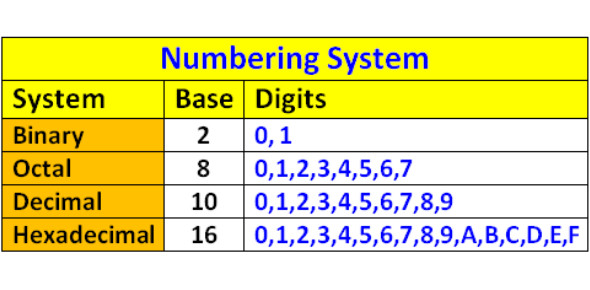# Number System Quiz For Binary, Decimal, And Hexadecimal

10 Questions | Total Attempts: 23090SettingsReady for our number system quiz for Binary, Decimal, and Hexadecimal? Mathematics is a unit that is concerned with numbers. Decimals are number systems that use the digits as of 1 to 9. A decimal system can be of different bases, such as 10 or 100. What do you know of the application of decimal systems? Study of Binary, Decimal & Hexadecimal Number Systems will explain it all. Go ahead with the quiz and learn everything you need to know.

• 1.
Convert the binary number 11001 to decimal. The answer is:
• A.

25

• B.

13

• C.

3

• 2.
Convert the decimal number 45 to binary:
• A.

11100

• B.

101101

• C.

10100

• 3.
Convert the hexadecimal number B2 to binary:
• A.

100011

• B.

11011

• C.

10110010

• 4.
Convert the binary number 11011 to hexadecimal:
• A.

1A

• B.

B1

• C.

1B

• 5.
Convert the decimal number 20 to hexadecimal:
• A.

14

• B.

11

• C.

1B

• 6.
Convert the hexadecimal number 2C to decimal:
• A.

3A

• B.

34

• C.

44

• 7.
Convert the binary number 10101100 to its decimal equivalent:
• A.

162

• B.

172

• C.

182

• D.

192

• 8.
Convert the decimal number 168 to binary equivalent:
• A.

10101110

• B.

01000100

• C.

10101000

• D.

11101000

• 9.
Convert the hexadecimal number 0x2301 to its binary equivalent:
• A.

0010001100000001

• B.

1111110011111110

• C.

1111000011001010

• D.

0000001111110000

• 10.
Covert the binary number 11010010 to a decimal number.
• A.

75

• B.

150

• C.

210

• D.

310

Related TopicsBack to top